# Setting up a DMD/SLM: Aberration effects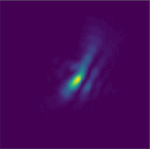Digital Micromirror Devices (DMDs) are amplitude only (binary) modulators, however, pretty much like liquid crystal modulators, they introduce some phase distortion. Practically, it means that if one illuminates the modulator with a plane wave, even when all the pixels are set to the same value, the wavefront shows phase distortions after reflection. That can be detrimental, especially when working in a plane conjugated with the Fourier plane of the DMD surface. Fortunately, using the Lee hologram method or the superpixel method, one can achieve phase modulation. I present here how to use Lee holograms to characterize and compensate for aberrations when using a DMD. This approach can also be applied for compensating for aberration effects in other types of Spatial Light Modulators, such as liquid crystal ones.

Let's consider a setup with a DMD using the Lee hologram method as presented in the corresponding tutorial page. We want to characterize the phase aberrations introduced by the DMD and compensate for them. To do so, we use a camera to measure the intensity image in the Fourier plane. With correct alignment and if one can neglect the aberrations of the other optical elements, one should obtain a nice Airy disk when displaying a disk on the SLM. I use in this experiment a DMD from Vialux (V-9601) with a Texas Instrument chip (DLP9600, 1920 by 1200 pixels with a 10.8-micron pitch) illuminated with a 633 nm laser. Displaying a 350 radius disk on the SLM results in the Point spread function (PSF) shown in Fig. 1.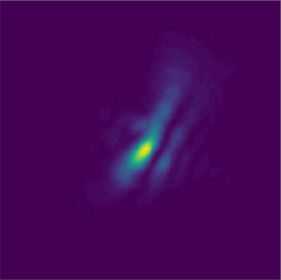Figure 1. Point spread function (PSF) of the aberrated system.

It shows significant aberrations. To check that the aberrations indeed come from the DMD, I replaced the DMD by a mirror and retrieved a nice symmetric PSF. We fall into a pretty common issue of adaptive optics, with the exception that the aberrations are not introduced by the propagating medium but by an imperfect optical element. If we make the assumption that the phase distortion comes from the fact that the surface of the DMD is not perfectly plane, we expect smooth and low-frequency phase aberrations, as we would have with atmospheric perturbations for instance.

Note that the distortion of the PSF depends on the radius on the illumination part of the DMD in this configuration. Indeed, increasing the illumination disk will result in using a higher numerical aperture and will also use a larger area on the DMD, where aberrations come from. When using a small part of the DMD (~100 to 200 pixels width), you may not even be sensitive to those aberrations.

Then, instead of doing a pixel by pixel optimization, as we like to do with complex media, we want to work in the basis of the Zernike polynomials, that we know is well suited for aberrations correction. The idea is to fix the number of Zernike polynomials we consider, and then we try different coefficients for each of them. We measure the output intensity profile for each configuration to find the coefficients that improve the best PSF.

### Generation of Zernike polynomials

To generate Zernike polynomials in Python, I use AOtools, a module in Python that gathers numerical tools for adaptive optics. In particular, the phaseFromZernikes(coeffs,N) generates an N by N phase mask from a list of coefficients coeffs for the different polynomials (see the documentation for more information).

Let's define a function to generate a complex phase mask

import numpy as np
from aotools.functions import phaseFromZernikes

'''
Generate a binary mask with value 1 inside a disk, 0 elsewhere
:param shape: list of integer, shape of the returned array
:center: list of integers, position of the center
:return: numpy array, the resulting binary mask
'''
if not center:
center = (shape//2,shape//2)
X,Y = np.meshgrid(np.arange(shape),np.arange(shape))

'''
Generate a complex phase mask from a vector containting the coefficient of the first Zernike polynoms.
:param DMD_resolution: list of integers, contains the resolution of the DMD, e.g. [1920,1200]
:param: integer, radius of the illumination disk on the DMD
:center: list of integers, contains the position of the center of the illumination disk
:center: list of float, the coefficient of the first Zernike polynoms
'''
# Generate a complex phase mask from the coefficients
# We want the amplitude to be 0 outside the disk, we fist generate a binary disk mask
# put the Zernik mask at the right position and multiply by the disk mask
mask = np.zeros(shape = shape, dtype=np.complex)
return mask

We can call the function for instance using:

complex_mask = complex_mask_from_zernike_coeff(shape = [1920,1200],
center = [1920//2,1200//2],
vec = [0.,0.,1.,2.,1.,5,0.75])

It generates a 2D complex array with only phase variations in a disk whose position and size are fixed by the user.

### Cost function

We want to improve the quality of the PSF, it implies maximizing the energy in a small area. As a cost function, we then take the ratio of the average intensity in a given disk (the signal) by the average energy elsewhere (the noise). This simple function can be written:

def get_cost(imgs, mask_radius = 8):
res = imgs.shape
X,Y = np.meshgrid(np.arange(res),np.arange(res))
# We generate a mask representing the disk we want to intensity to be concentrated in
cost = signal/noise
return cost

### Optimization

First, we fix the number of polynomials we want to test. For each polynomial, we test different values and record the corresponding images. We keep the value that gives the best cost function and move to the next coefficient. I do not give an example code as the implementation depends upon the specific hardware one is using.

### Results

After optimization, I obtain a phase mask that gives me the corrected PSF shown in Fig 2. We retrieve a nice PSF looking like an Airy disk.

###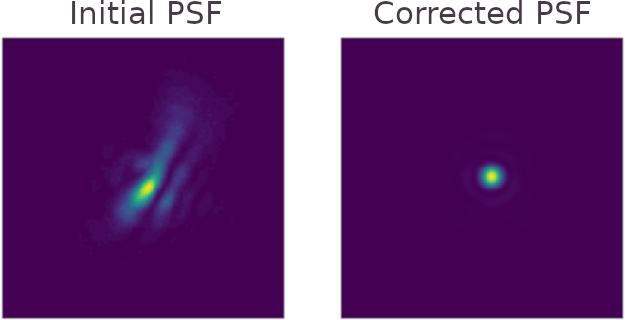Figure 2. Corrected PSF (right) and initial PSF (left).

For this experiment, I optimized 14 Zernike coefficients. I display the optimal values in Fig 3. It is important to make a few remarks:

• the first Zernike coefficient is irrelevant because it corresponds to a global phase shift (piston) that does not affect the PSF,
• the second and third coefficients are phase shifts, corresponding to lateral shifts of the PSF. As we were already centered around the maximum of the PSFD, the best values found were zero,
• the optimal values for the last 6 coefficients are also equal to zero, which means that we were right in making the assumption that the distortion introduced by the aberration is quite low-frequency. It is then not necessary to use more coefficients than 8 in this case. Be aware that the number of polynomials to take into account depends on the radius of the illumination. The bigger the aperture, the greater the effect of the perturbations.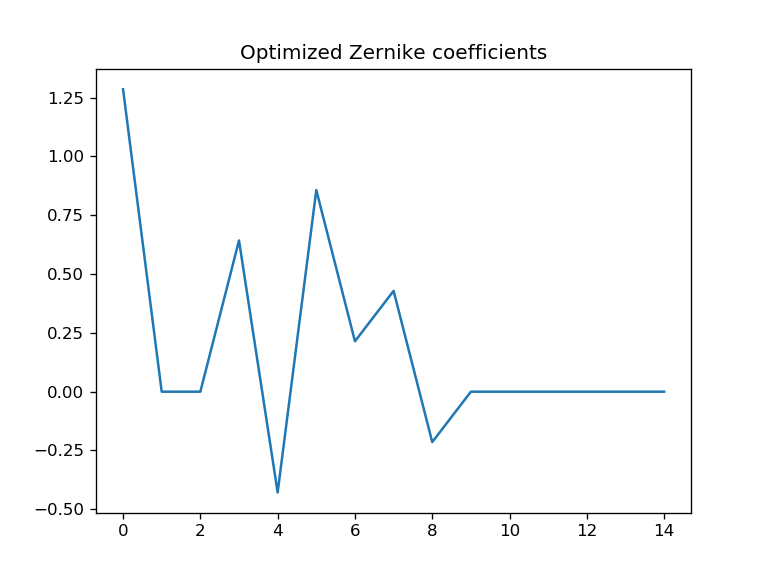Figure 3. Optimized coefficients for the Zernike polynomials.

I show in Fig. 4 the aspect of the phase mask that compensates for the effect of the aberrations. It corresponds to the phase conjugation of the phase distortion introduced by the imperfect flatness of the DMD.

Figure 4. Optimal phase pattern corresponding to the phase conjugation of the phase distortion introduced by the aberrations.

Finally, I show in  Fig 5. the corresponding binary amplitude Lee Hologram that I display on the DMD to obtain the corrected PSF shown in Fig 2.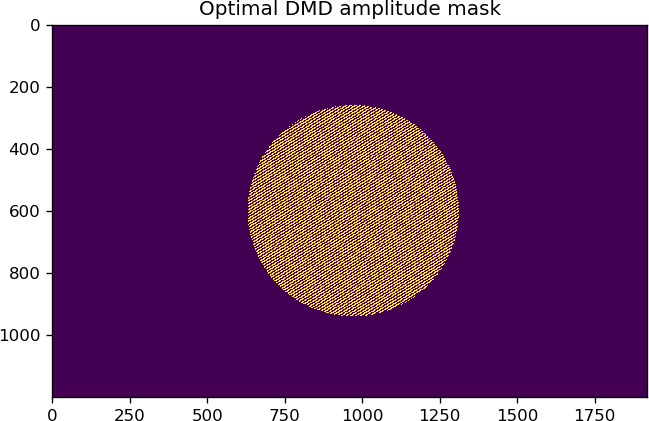Figure 5. Binary Lee Hologram pattern corresponding to the optimal phase mask.

Created by sebastien.popoff on 07/08/2019